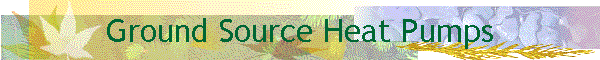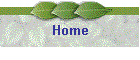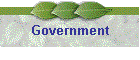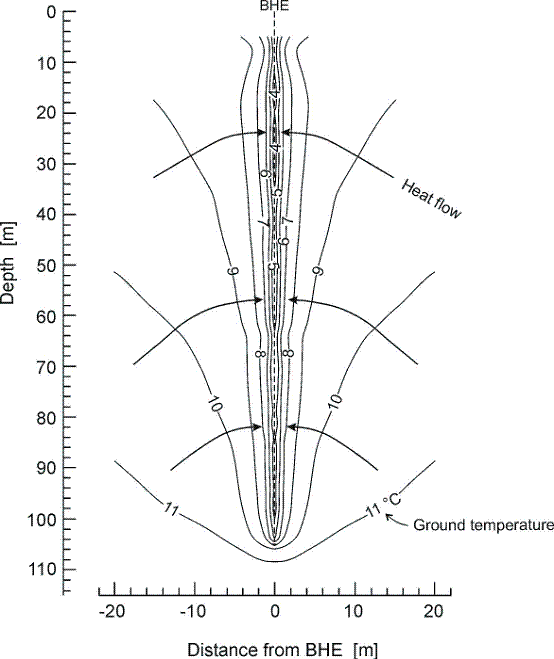We are in the process of fitting a ground source heat pump to our house using vertical bore holes (or boreholes) as the ground source.

This page will document the process and results, and also some rough calculations I have made to convince myself that the installers are doing the correct thing. These calculations give easily reasons why some installation techniques are counter-intuitive (why multiple ground loops should be connected in parallel not series), and shows why some quite minor tweaks to the installation make a large difference in the efficiency of the system (putting spacers between the pipes in a loop).

We will be having three 60m boreholes, so all the calculations assume 180m of borehole total (and thus 360m of pipe in the U-tubes).

## How much heat is in how much rock?

In order to make the pump as efficient as possible we want to extract as much heat as fast as possible from the ground loop, but we might be worried that if we draw out the heat to fast it will be unsustainable and we will "run out" of heat. Certainly over a long time the output will drop as the system reaches equilibrium, but is a major removal of heat likely?

This paper suggests that for "limestone (massive)" you can safely extract up to 45-60 W/m of borehole for 2400hours/year (and for clay 30-40 W/m). They use double U-tubes for this.

For 180m of boreholes this equates to 45 * 180 /1000 = 8.1KW when on, or 19440 KWHr / year when on for 2400 hours per year. Given that some of the heat will come from the electricity, this gives us 8.1 * (COP/COP-1) =  11.3 KW (assuming a COP of 3.5). Which is the rated size of the heat pump we are getting. So this looks plausible, but could we do more?

Now let's look at how much heat the rock stores - I'll assume that we've got a three 60m vertical bore holes through chalk/limestone.

#### How much will the temperature of the holes drop assuming we look at a cylinder of rock 5m in diameter, 60m deep for each borehole?

Specific Heat (volumetric heat actually) of rock is around 2 MJ.m-3.K-1 (a Watt = 1 Joule per second)

Temperature rise  = Energy / (volume * volumetric heat)

Assuming 11KW heat pump,  COP 3.5,  230 days per year, 18 hours per day

1 years heat = 11KW * 230 * 18 * (2.5/3.5) = 32528 KWHr = 32528 * 3600 J = 117101 MJoules...

Let's see what temperature drop this would get in three 60m boreholes assuming it affects a 5m diameter cylinder around each:

Volume = 3*60*(5/2)2*pi = 3540 m3

Temperature drop = 117101 *1000000 / (3540 * 2000000) =

Specific Heats

The thermal conductivity of clay varies from 0.5-1.4 W.m-1.K-1 for dry (5%) clay and from 0.7-1.9 W.m-1.K-1 for wet (20%) clay.

Thermal diffusivity of clay =0.033-0.060 and 0.028 to 0.059 M2/day

Diffusivity = conductivity/volumetric heat capacity.

Limestone (chalk) varies from 2.4 - 3.8 W/M/K

Limestone Specific heat 920 J.Kg-1.K-1

Limestone density= 2400-2800 Kg/m3

#### Temperature drop = 17 degrees/year

Which is quite a lot! Which explains why there is a limit to how much heat is extracted per year as you are reliant on the heat transfer rate from the ground around our cylinder of rock to replenish the energy we are consuming.

How big a cylinder do we need to only drop a degree? Well we need 17 times more volume, so 4.1 times bigger diameter, so a 20.5m diameter cylinder!

#### Extraction rate by rise in temperature on input water

The flow rate of the heat pump is 0.58 litres of heat transfer fluid per second. The specific heat of the fluid is ?

Water is 4184 J.litre-1.K-1

Ethylene Glycol is 2470 J.litre-1.K-1

http://www.geo4va.vt.edu/A2/A2.htm

This one gives the amount of watts you can safely get from 1m of pipe per year, and talks about multiple boreholes: